×#### Thank you for registering.

One of our academic counsellors will contact you within 1 working day.

Click to Chat

1800-1023-196

+91-120-4616500

CART 0

• 0

MY CART (5)

Use Coupon: CART20 and get 20% off on all online Study Material

ITEM
DETAILS
MRP
DISCOUNT
FINAL PRICE
Total Price: Rs.

There are no items in this cart.
Continue Shopping• Complete JEE Main/Advanced Course and Test Series
• OFFERED PRICE: Rs. 15,900
• View Details

```Revision Notes on Force and Laws of Motion

How does an object start moving?

We need to put some effort to make a stationary object move, For Example, a push, a hit or a pull.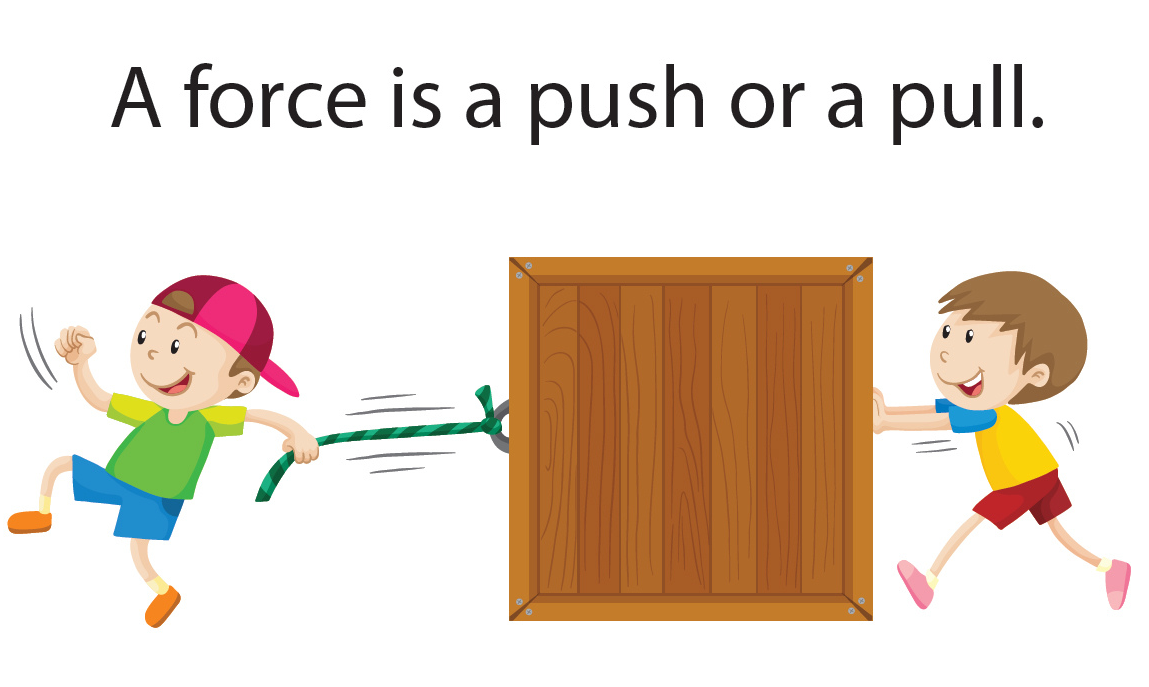Fig.1- How does an object move

What is a force?

Whenever we push or pull an object a force acts upon them and makes them move from one place to another. Hence, force can –

initiate motion in a motionless object

change (increase or decrease) the velocity of the moving object

alter the direction of a moving object

change the shape and size of an objectFig.2 - Effects of Force

Balanced and Unbalanced Forces

Balanced Forces –

When equal amount of forces are applied on an object from different directions such that they cancel out each other

They do not change the state of rest or motion of an object

They may change the shape and size of an object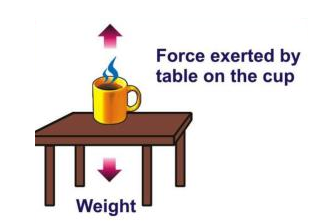Fig.3- Balanced Forces

Unbalanced Force –

When forces applied to an object are of different magnitude(or not in opposite directions so as to cancel)

They can alter state of rest or motion of an object

They can cause acceleration in an object

They can change the shape and size of an object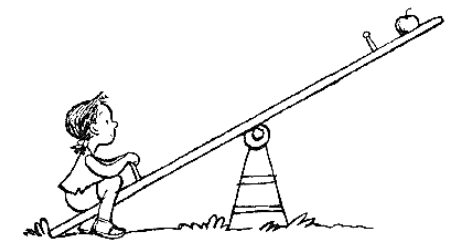Fig.4 – Unbalanced Forces

What is the force of friction?

It is a force extended when two surfaces are in contact with each other. It always acts in a direction opposite to the direction of motion of the object.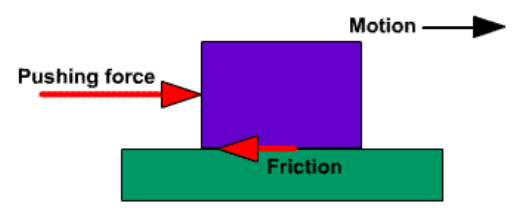Fig. 5 – The force of Friction

First Law of Motion

Galileo’s Observation

He observed the motion of objects on an inclined plane.

When a marble is rolled down an inclined plane its velocity increases.

Galileo’s Arguments

When a marble is rolled down from the left – It will go up on the opposite side up to the same height at which it is dropped down.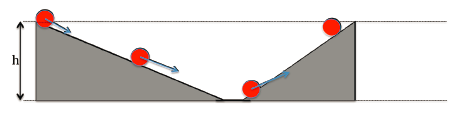If the inclination of planes is equal – The marble would travel equal distances while climbing up as travelled while rolling down.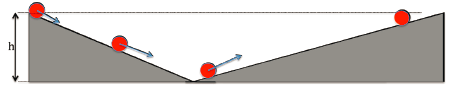If we decrease the angle of inclination of the right plane – The marble would travel further until it reaches its original height.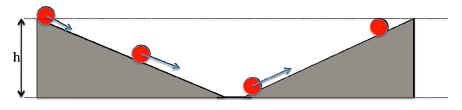If the right side plane is made flat – Marble would travel forever to achieve the same height.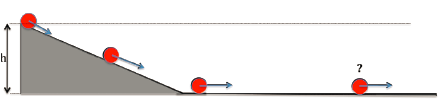Galileo's Inference

We need an unbalanced force to change the motion of the marble but no force is required when the marble is moving uniformly. In other words, objects move at a constant speed if no force acts upon them.

Based on Galileo’s ideas Newton presented the three Laws of Motion

First law of motion or The Law of Inertia

Whether an object is moving uniformly on a straight path or is at rest, its state would not change until and unless an external force is applied on to it.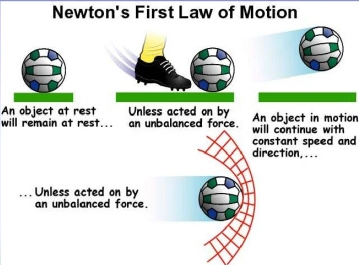Hence, we can say that objects oppose a change in their state of motion or rest. This tendency of objects to remain in the state of rest or to keep moving uniformly is called Inertia.

Examples of Inertia

We fall back when a vehicle starts moving in the forward direction because our body is in the rest state and it opposes the motion of the vehicle.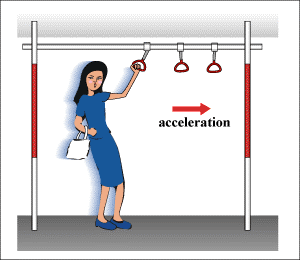We fall forward when brakes are applied in a car because our body opposite the change of state of motion to rest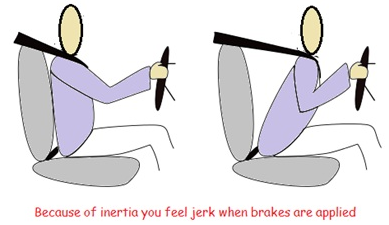Inertia and Mass

The inertia of an object is dependent upon its mass.

Lighter objects have less inertia, that is, they can easily change their state of rest or motion.

Hence ‘Mass’ is called a measure of the inertia of an object.

Consider the image given below; it is easier for a person to push the bucket that is empty rather than the one that is filled with sand. This is because the mass of an empty bucket is less than that of the bucket filled with sand.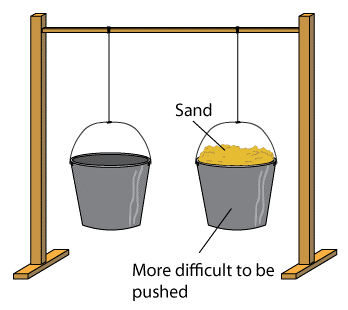The Second Law of Motion

The impact produced by a moving object depends upon its mass and velocity.

For Example, a small bullet fired at a high velocity can kill a person.

Momentum – The product of mass and velocity is called Momentum.

It is a vector quantity. Its direction is same as that of the object’s velocity.

Denoted by – p

SI unit – kg metre per second

p = mv,

where m is the mass of the object,

v is the velocity of the object

The momentum of a stationary object –

Let the mass of a stationary object be ‘m’,

Let the velocity of a stationary object be ‘v’,

The stationary object has no velocity, so v = 0,

Therefore, p = m*v = m*0 = 0

So, the momentum of a stationary object is zero.

We know that the velocity of an object can be changed by applying an unbalanced force on to it. Similarly, the momentum of an object can change by applying an unbalanced force.

According to the second law of motion –

The rate of change of momentum of an object is directly proportional to the applied unbalanced force on the object in the direction of the force.

For Example –

A cricketer when catches a ball pulls his hands in the backward direction to give some time to decrease the velocity of the ball. As the acceleration of the ball decreases the force exerted on catching the moving ball also decreases. If the cricketer would try to stop a moving ball suddenly he would have to apply larger force.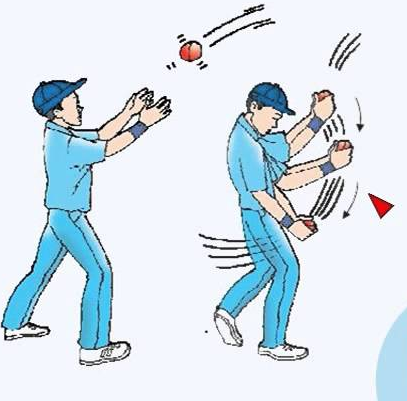Mathematical Formulation of the Second Law of Motion

Based on the definition of the second law of motion, we can infer that -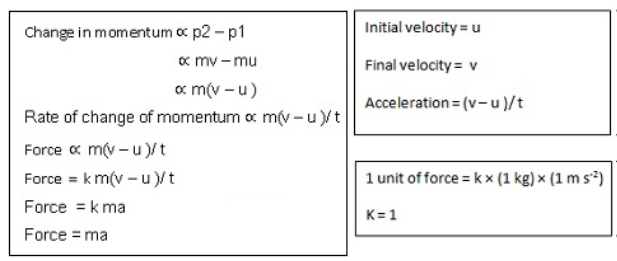Therefore, with help of the second law of motion we can evaluate the amount of force that is being exerted on any object. From the formula stated above, we can see that the force is directly proportional to acceleration. So the acceleration of an object can change depending upon the change in force applied.

Force = ma

SI Unit: kg-ms-2 or N (Newton)

The Third Law of Motion

Action and Reaction Forces

Two forces acting from opposite directions are called Action and Reaction Forces.

For Example, a ball when hits the ground (action) bounces back with a certain force reaction.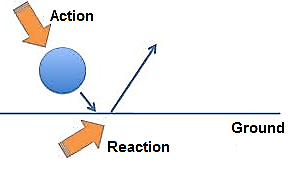Fig. 15 - Action and Reaction Forces

The Third Law of Motion States that –

When an object exerts a force on another object, the second object instantly exerts a force back onto the first object. These forces are always equal in magnitude but opposite in direction. These forces act on two different objects always.

Or in other words, every action has an equal and opposite reaction.

The magnitudes of forces acting upon the objects are same but the acceleration produced in them may or may not be the same because the objects can differ in masses.

For Example, when a bullet is fired from a gun, the gun only moves a little backwards (recoils) while the bullet can travel a large distance. This is because of the difference in the mass of the bullet and the gun.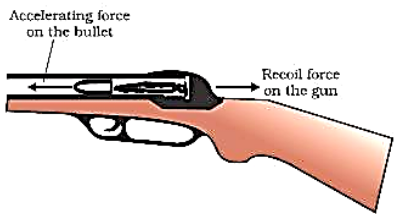Conservation of Momentum

As per the law of conservation of momentum, the sum of momenta of two objects before the collision and after collision remains the same given that no external unbalanced force acts upon them. In another way, collision conserves the total momentum of two objects.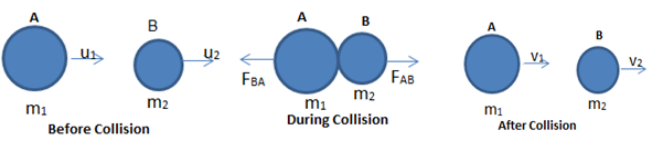Consider the figure given above. Two balls A and B having a certain initial velocities collide with each other. Conditions before the collision-

There is no unbalanced force acting upon them

The initial velocity of A is greater than initial velocity of B

The figure below explains how the momentum of the balls is conserved after the collision.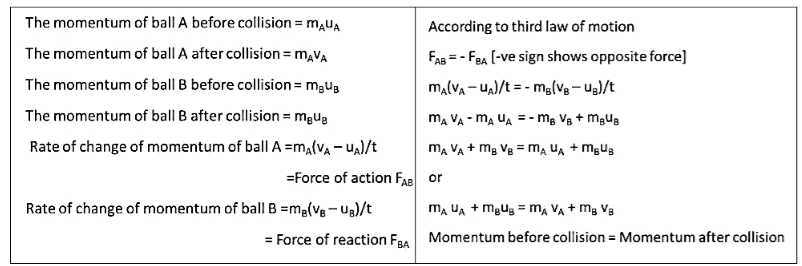Fig.18 – Conservation of Momentum

They are considered as the fundamental laws in physics.

They are based on observations and experiments.

They cannot be proved but can be verified or disproved with the help of experiments.

A single experiment is enough to disprove a law, while a single experiment is not enough to prove the same.

It requires a large number of experiments to prove the law.

The law of conservation of momentum was formulated 300 years ago.

There is no single situation present until now that disproves this law.

Other laws of conservation are – law of conservation of energy, the law of conservation of angular momentum, the law of conservation of charge.

```### Course Features

• 728 Video Lectures
• Revision Notes
• Previous Year Papers
• Mind Map
• Study Planner
• NCERT Solutions
• Discussion Forum
• Test paper with Video Solution